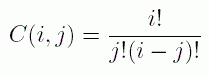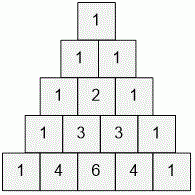Competitions

# Combination

How many numbers are divisible by the prime number p in the first n rows of Pascal Triangle? In other words, find the number of pairs (j, i) (0ji < n) so that C(i, j) is divisible by p. Here#### Input

The first line contains two integer numbers n, p (1n107, 3p100).

#### Output

Time limit 1 second
Memory limit 128 MiB
Input example #1
5 3


Output example #1
3

Input example #2
100 7

Output example #2
2689
Source 2014 KBTU Open, Spring Kazakhstan, Almaty, April 20, Problem B